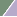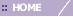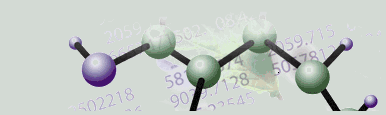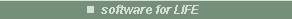# Normalization Overview

Overview

In GeneLinker™ the term normalization is used to describe scaling, translation, or any other numerical transformation of the data besides filtering. These transformations fall into three broad categories:

• You may need to correct for non-biological variations between different samples. For example, unintentional differences in hybridization procedures or between microarray chip manufacturing batches may cause systematic differences between samples. Normalizations which can help correct these sources of variation include mean scaling, median scaling, linear regression and control gene normalizations.

• Two-color data must be merged into ratios, and dye biases can also be corrected for at the same time.

• If you are going on to study the data by clustering, you may need to put different genes on a single scale of variation. Normalizations which may accomplish this include logarithm, standardization, division by maximum and scaling between 0 and 1.

Any number of these normalizations can be applied to dataset in succession. For instance, it may be appropriate to scale samples to correct for non-biological variations, and then place genes on a common scale before clustering, association mining or supervised learning takes place.

Techniques for Correcting Non-Biological Variation Between Samples

• Linear Regression: This procedure scales the values relative to a baseline sample so that the best-fit slope of each sample is equivalent. All genes can be fitted, or only a user-selected set of 'housekeeping' genes.

• Division by Central Tendency (Mean): This procedure scales the expression values so that all samples have a common mean.

• Division by Central Tendency (Median): This procedure scales the expression values so that all samples have a common median.

• Positive and Negative Control Genes: In some experiments there may be one or more control genes whose values are expected to be constant. With multiple controls, the median or mean is calculated over all of the controls.

• Normalization relative to negative controls subtracts the median or mean of the controls within the sample. Negative control genes are understood to be absent or below a detection threshold.

• Normalization relative to positive controls divides each sample by the mean or median of the controls. Positive control genes are understood to be present in constant abundance in all samples.

• Lowess: The log-ratio expression values are adjusted by a locally-weighted linear regression on each sample to account for intensity-dependent dye bias.

• Logarithm: Gene expression values are replaced with the logarithm of their values. Taking the logarithm equalizes the influence of up- and down-regulated genes in ratio experiments.

• Subtraction of Central Tendency: This procedure transforms the expression values such that all samples have zero mean or median.

The Lowess normalization automatically merges the treatment and control channels into adjusted ratios. Any other operation on a two-color table automatically uses the unadjusted ratios.

Note: Lowess is the only normalization option for incomplete two-color datasets.

Techniques for Placing Different Genes on a Similar Scale

• Logarithm: Gene expression values are replaced with the logarithm of their values. In non-ratio experiments, taking the logarithm reduces the influence of high-abundance genes in comparison to low-abundance genes.

• Divide by Maximum: Gene expression values are scaled such that the largest value for each gene becomes one.

• Scaling Between 0 and 1: Gene expression values are scaled such that the smallest value for each gene becomes zero and the largest value becomes one. Also known as Min-Max Normalization.

• Standardize: Gene expression values are scaled such that each gene has an average of zero and a standard deviation of one.

Related Topics:

Filtering Overview

Clustering Overview